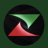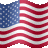# Sine Wave Support & Resistance

T

##### Active member
@Nomak not sure if you felt up to it, but I had this in my archives from tradingview. I think its similar to what @thebewb has on his chart if you wish to code it over (or anyone else that feels like they may want it. Linking both the indicator and the code here for you. https://www.tradingview.com/script/l3fFpSkr-Hilbert-Sine-Wave-Support-and-Resistance/

Code:
``````//@version=2
study("Hilbert Sine Wave Support and Resistance", "Sine Wave SR", overlay=true)
Price = close
alpha = 0.07
Smooth = (Price+2*Price+2*Price+Price)/6
Cycle = (1-.5*alpha)*(1-.5*alpha)*(Smooth-2*Smooth+Smooth)+2*(1-alpha)*nz(Cycle)-(1-alpha)*(1-alpha)*nz(Cycle)
Q1=(.0962*Cycle+.5769*Cycle-.5769*Cycle-.0962*Cycle)*(.5+.08*nz(InstPeriod))
I1 = Cycle
DeltaPhase = iff(Q1 != 0 and Q1!= 0, (I1/Q1-I1/Q1)/(1+I1*I1/(Q1*Q1)), na)
DeltaPhase := iff(DeltaPhase < 0.1, 0.1, DeltaPhase)
DeltaPhase := iff(DeltaPhase > 1.1, 1.1, DeltaPhase)
MedianDelta = percentile_nearest_rank(DeltaPhase,5, 50)
DC = iff(MedianDelta == 0, 15, 6.28318/MedianDelta + 0.5)
InstPeriod=.33*DC+.67*nz(InstPeriod)
Value1 = .15*InstPeriod+.85*nz(Value1)
DCPeriod = floor(Value1)
RealPart = 0
ImagPart = 0
for count = 0 to DCPeriod - 1
RealPart := RealPart + sin(6.28318 * count / DCPeriod) * (Cycle[count])
ImagPart := ImagPart + cos(6.28318 * count / DCPeriod) * (Cycle[count])
DCPhase = iff(abs(ImagPart) > 0.001, atan(RealPart / ImagPart), na)
DCPhase := iff(abs(ImagPart) <= 0.001, 1.572963*sign(RealPart), DCPhase)
DCPhase := DCPhase + 1.572963
DCPhase := iff(ImagPart < 0, DCPhase + 3.1415926, DCPhase)
DCPhase := iff(DCPhase > 5.49778705, DCPhase - 6.28318, DCPhase)
Sine = sin(DCPhase)

// Drawing Lines:
drawingsupport = nz(drawingsupport)
currdotvalue = nz(currdotvalue)
if (LeadSine[floor(DCPeriod/8)] <= Sine[floor(DCPeriod/8)] and drawingsupport == 1)
currdotvalue := high*1.01
drawingsupport := 0
if (LeadSine[floor(DCPeriod/8)] > Sine[floor(DCPeriod/8)] and drawingsupport == 0)
currdotvalue := low*0.99
drawingsupport := 1
currdotcolor = iff(drawingsupport == 0, green, red)
plot(currdotvalue, style=circles, linewidth=2, color=currdotcolor)``````

•L

#### lance

##### New member
Hi -did you get any traction on this? I'd like to get the indicator for TOS as well.

L

#### lance

##### New member
Hi - is there a thinkscript study for the sine wave S/R indicator?

TBetter Sine Wave Questions 0Major And Minor Wave Lines / Price Channel Labels for ThinkorSwim Indicators 16EWO (Elliot Wave Oscilator) Questions 15